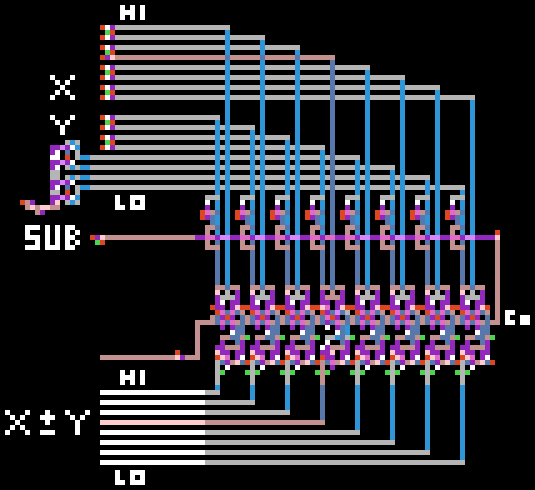# ALU in boilerplate

I've been taking a break from serious programming to spend some time messing around with boilerplate again.

Its a hunk of boilerplate logic which can add or subtract (!!) any two numbers.The two numbers come in on the top left. They're represented in binary with the high bit at the top. One of the numbers is fixed at 16 and the other is setup to cycle through the values of 0-15.

The ALU can either add or subtract - I've set it to subtract here. I only really have logic to add, but `X - Y` is the same as `X + -Y`, which in turn is the same as `X + ~Y + 1`. The little jackets on the wires in the middle of the diagram are inverters. The subtract flag selects between the inverted values and the normal values. Then I'm passing `X` and `~Y` into the 8 bit adder (!!) and adding 1 via the carry-in gate on the right.

The result is the numbers from 16 down to 1 coming out. Isn't that awesome! :D